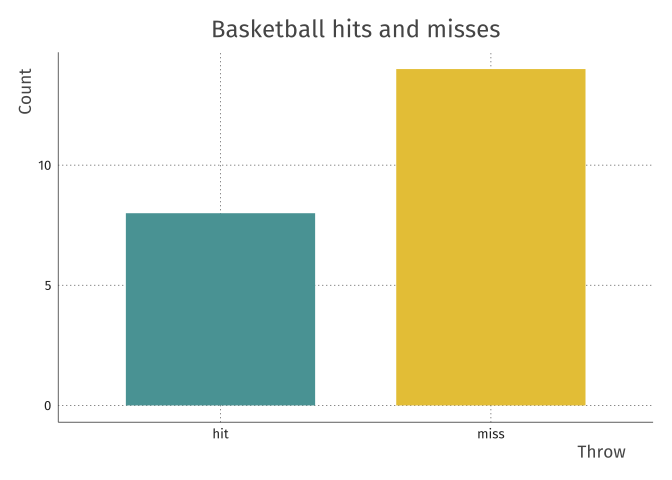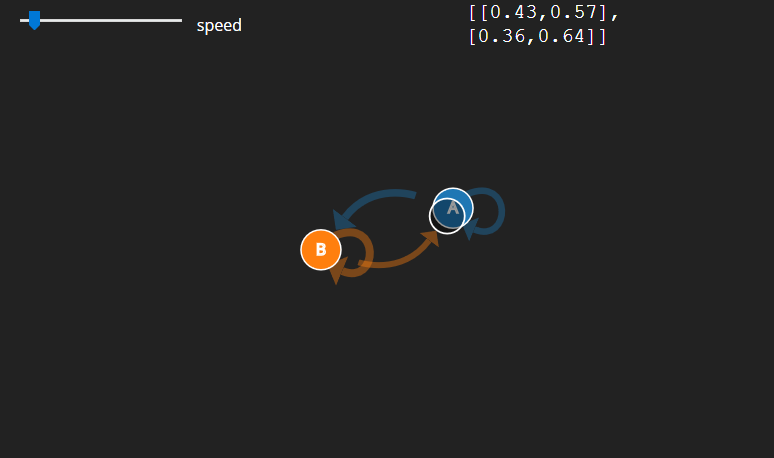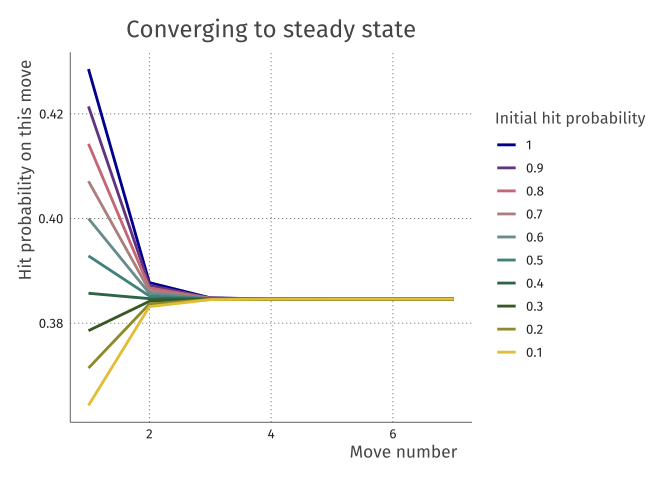## Introduction

I was on holiday and had to wait a while. Fortunately, close to where I was sitting there was a kid playing basketball all by himself. Of course I had to record how many hits and misses he made, to keep it as a nice dataset for further analysis.

Here is the dataset:

basket <- c("miss","miss","miss","hit","miss","hit","miss", "miss",
"hit","hit","miss","miss","miss","miss", "miss","miss",
"miss","hit","hit","hit","miss","hit")

## What can we do with this?

Firstly, we can describe how often the kid manages to score a point:So it looks like the kid misses a bit more than he hits. But there is much more information in this dataset: besides whether the kid hits or misses, we can say something about the sequence of these events.

## Transition matrix

From a sequence of observations, it is possible to construct a transition matrix

$\begin{bmatrix} A & B \\ C & D \end{bmatrix}$ Where the elements indicate the probability that:

• $$A$$: a hit is followed by another hit
• $$B$$: a hit is followed by a miss!
• $$C$$: a miss is followed by a hit
• $$D$$: a miss is followed by another miss

In other words, $$B$$ and $$C$$ indicate how likely it is to transition from hit to miss and vice versa, whereas $$A$$ and $$C$$ indicate how likely it is to stay in the same state (transition to self).

If we don’t know the true probabilities, we can enter the observed probabilities into the matrix. Here is an R function for generating the transition matrix from the data vector of before:

transMat <- function(x, prob = TRUE) {
X <- t(as.matrix(x))
tt <- table( c(X[,-ncol(X)]), c(X[,-1]) )
if (prob) tt <- tt / rowSums(tt)
tt
}

print(transitionMatrix, digits = 2)
##
##         hit miss
##   hit  0.43 0.57
##   miss 0.36 0.64

## Markov chain

This transition matrix completely defines the 2-state markov chain. Assuming these probabilities are stable, we can now generate processes just like the one we observed. And we can nicely visualise it using this markov chain generator post by setosa. Go to this link to play around!If we assume this chain is stable over time, there is another nice property. Irrespective of the initial probabilities of hitting or missing that we choose, after a few steps of the markov process, the probability of hitting the basket already converges to the steady state:

## Warning: as.tibble() is deprecated, use as_tibble() (but mind the new semantics).
## This warning is displayed once per session.In general, we can calculate the steady state easily from the transition matrix:

someBigNumber <- 1000
diag(transitionMatrix %^% someBigNumber)
##       hit      miss
## 0.3846154 0.6153846

Note that this leads to a similar but different result than simply counting the probability of hitting or missing as a table, as we did in the first figure:

table(basket)/length(basket)
## basket
##       hit      miss
## 0.3636364 0.6363636

# Questions

• What should we trust here? The naÃ¯ve probabilities or the markov steady state?
• Which assumptions lead to this discrepancy?
• Does the transition matrix really contain more relevant information about this process than the observed hit rate?